# On the Communication Complexity of SFE with Long

• Slides: 33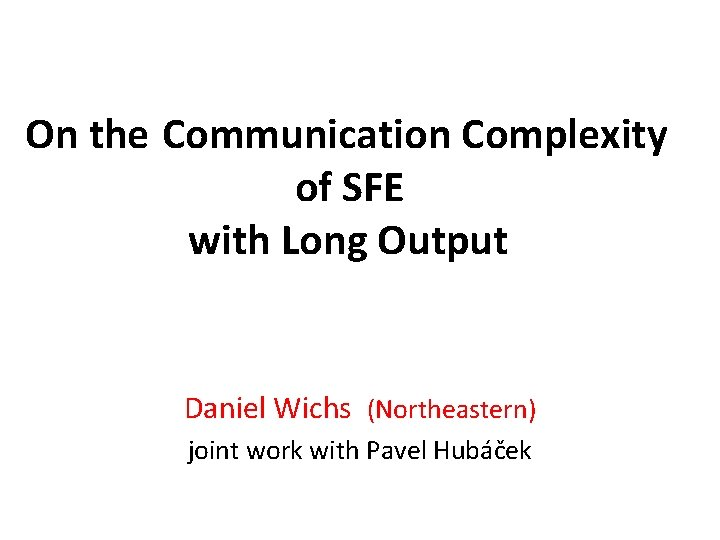On the Communication Complexity of SFE with Long Output Daniel Wichs (Northeastern) joint work with Pavel Hubáček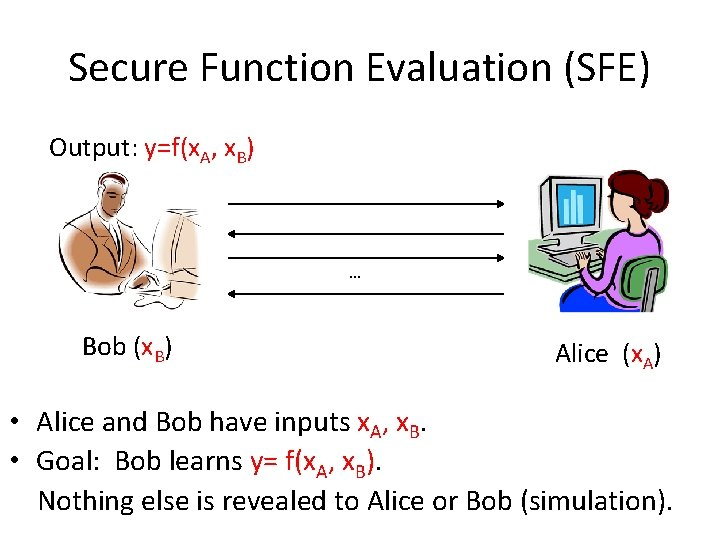Secure Function Evaluation (SFE) Output: y=f(x. A, x. B) … Bob (x. B) Alice (x. A) • Alice and Bob have inputs x. A, x. B. • Goal: Bob learns y= f(x. A, x. B). Nothing else is revealed to Alice or Bob (simulation).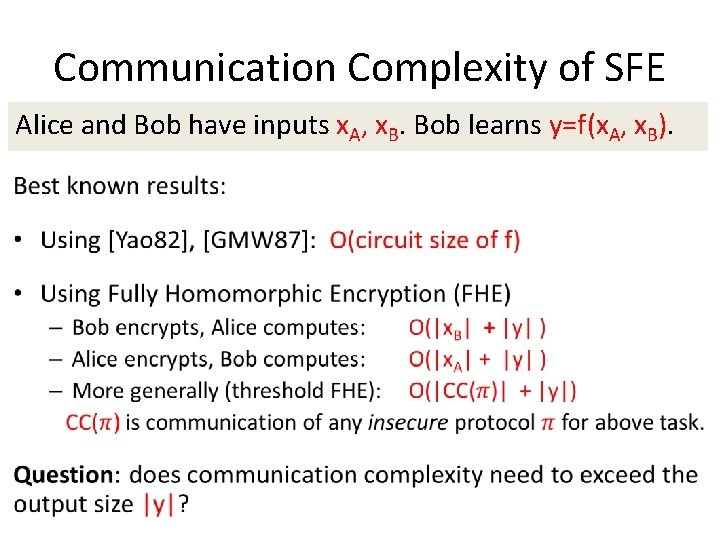Communication Complexity of SFE Alice and Bob have inputs x. A, x. B. Bob learns y=f(x. A, x. B).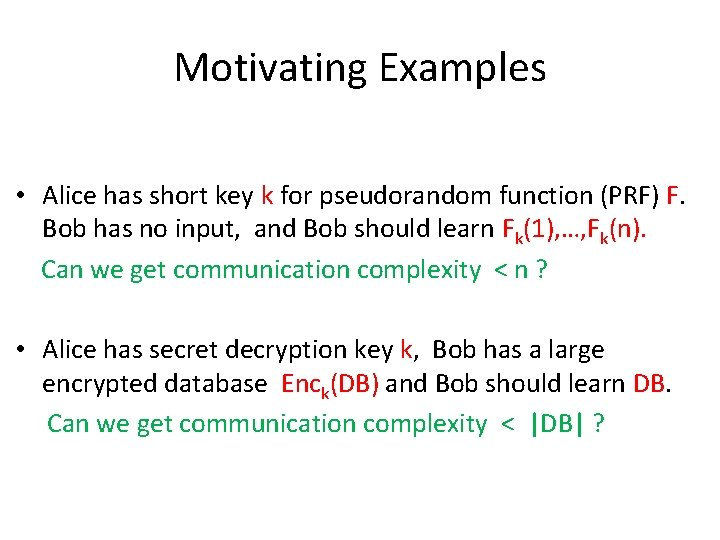Motivating Examples • Alice has short key k for pseudorandom function (PRF) F. Bob has no input, and Bob should learn Fk(1), …, Fk(n). Can we get communication complexity < n ? • Alice has secret decryption key k, Bob has a large encrypted database Enck(DB) and Bob should learn DB. Can we get communication complexity < |DB| ?Overview of Our Results Negative: In any general SFE scheme in the fully malicious setting, the communication complexity must exceed output size. • Extends to “honest-but-deterministic” setting: corrupted party follows protocol but does not use randomness (random tape = 0*). Positive: Construct a general SFE scheme in the honestbut-curious setting whose communication matches the best insecure protocol (independent of output size). • Relies on heavy hammers: indistinguishability obfuscation and FHE.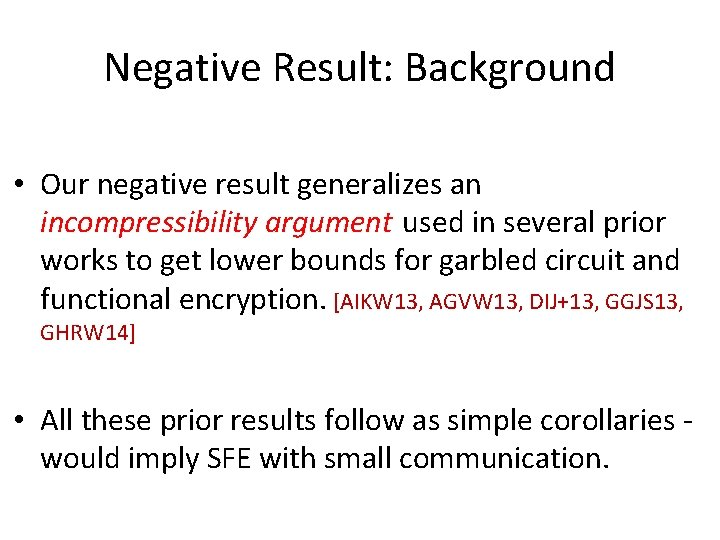Negative Result: Background • Our negative result generalizes an incompressibility argument used in several prior works to get lower bounds for garbled circuit and functional encryption. [AIKW 13, AGVW 13, DIJ+13, GGJS 13, GHRW 14] • All these prior results follow as simple corollaries - would imply SFE with small communication.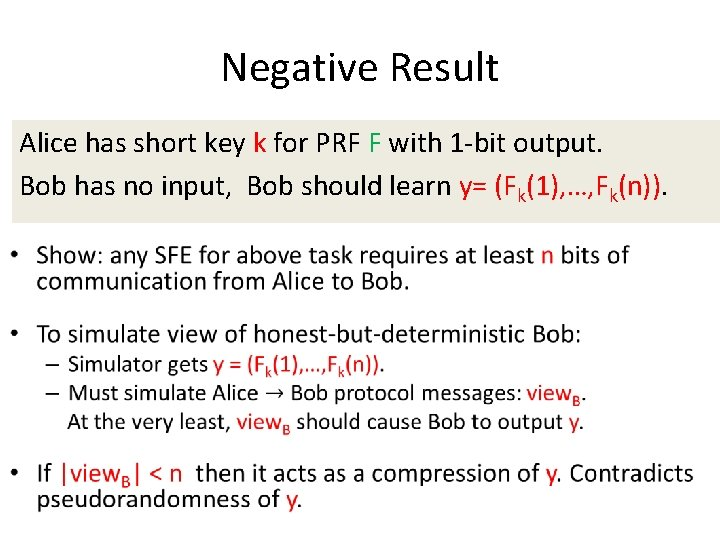Negative Result Alice has short key k for PRF F with 1 -bit output. Bob has no input, Bob should learn y= (Fk(1), …, Fk(n)).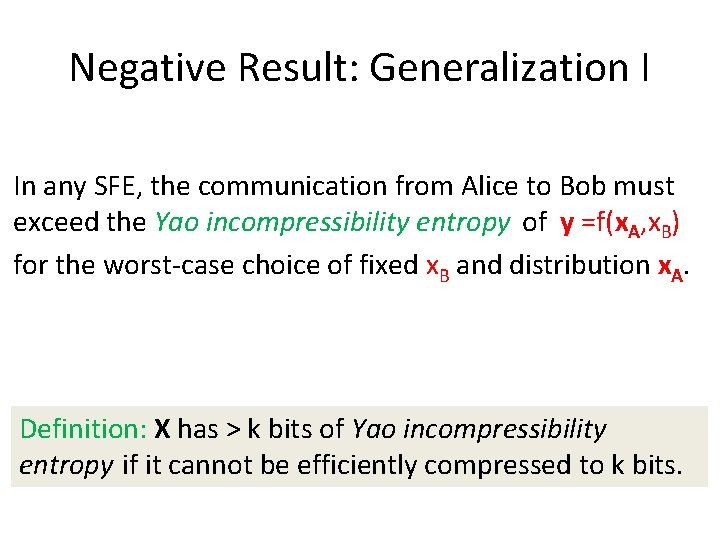Negative Result: Generalization I In any SFE, the communication from Alice to Bob must exceed the Yao incompressibility entropy of y =f(x. A, x. B) for the worst-case choice of fixed x. B and distribution x. A. Definition: X has > k bits of Yao incompressibility entropy if it cannot be efficiently compressed to k bits.Negative Result: Generalization II Can we have an offline/online* protocol with small online communication, independent of output size? *offline phase executed before parties know their inputs. Not if the offline phase has to be simulated first, before simulator knows input/output of corrupted party. • e. g. , inputs are chosen adaptively after offline phase. ( Yes otherwise: can use Yao garbled circuits. )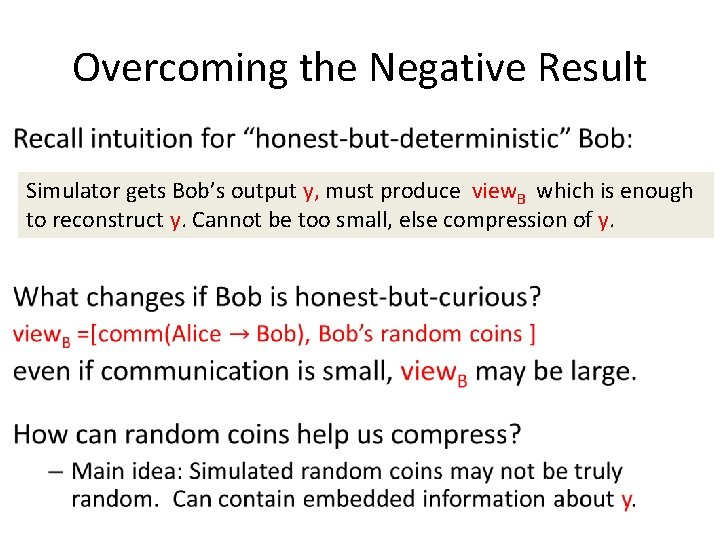Overcoming the Negative Result • Simulator gets Bob’s output y, must produce view. B which is enough to reconstruct y. Cannot be too small, else compression of y.Positive Result: Simplified Goal Alice has short key k for PRF F with 1 -bit output. Bob has no input, Bob should learn y= (Fk(1), …, Fk(n)). • As a start let’s focus on above task, later generalize to any SFE. • Goal: – Security against honest-but-curious Bob. – Communication complexity << n.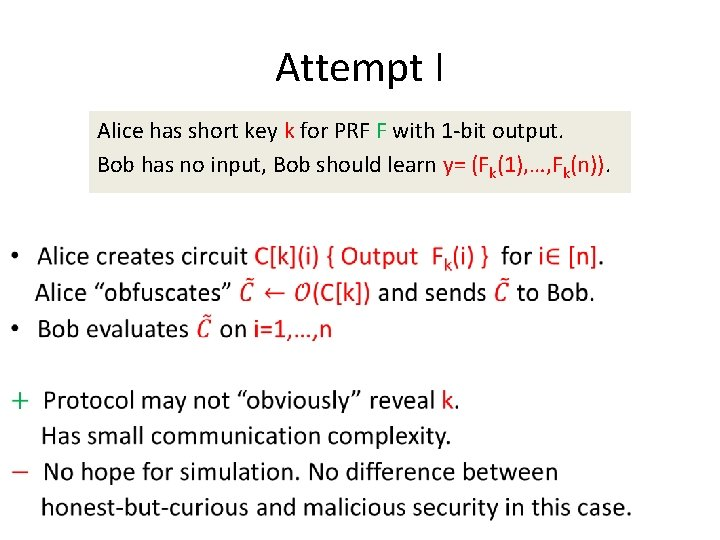Attempt I • Alice has short key k for PRF F with 1 -bit output. Bob has no input, Bob should learn y= (Fk(1), …, Fk(n)).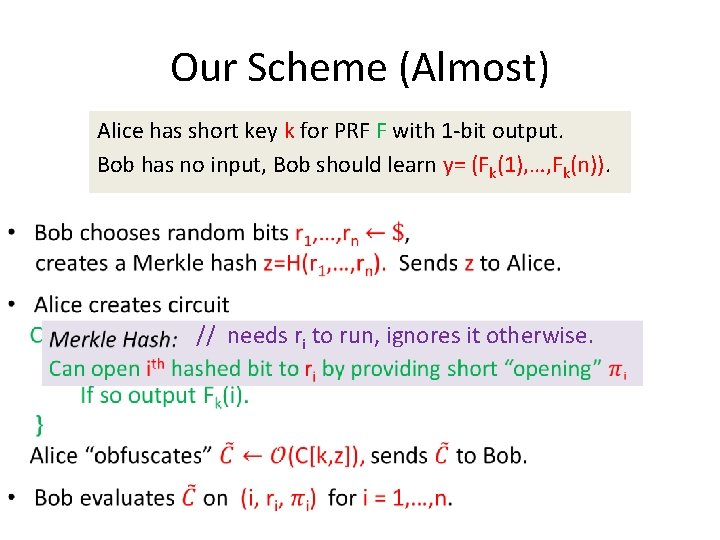Our Scheme (Almost) • Alice has short key k for PRF F with 1 -bit output. Bob has no input, Bob should learn y= (Fk(1), …, Fk(n)). // needs ri to run, ignores it otherwise.Protocol Simulation •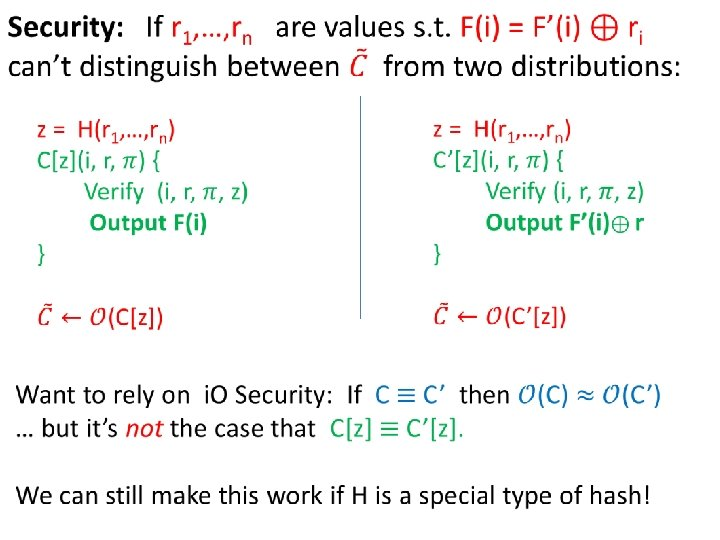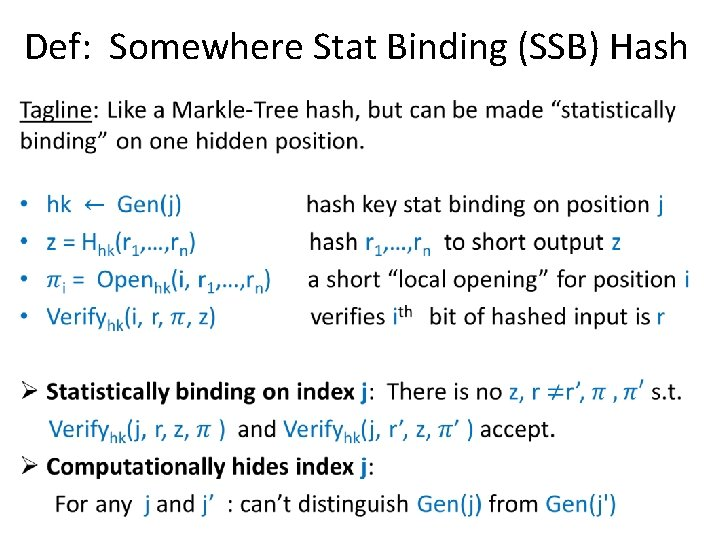Def: Somewhere Stat Binding (SSB) Hash •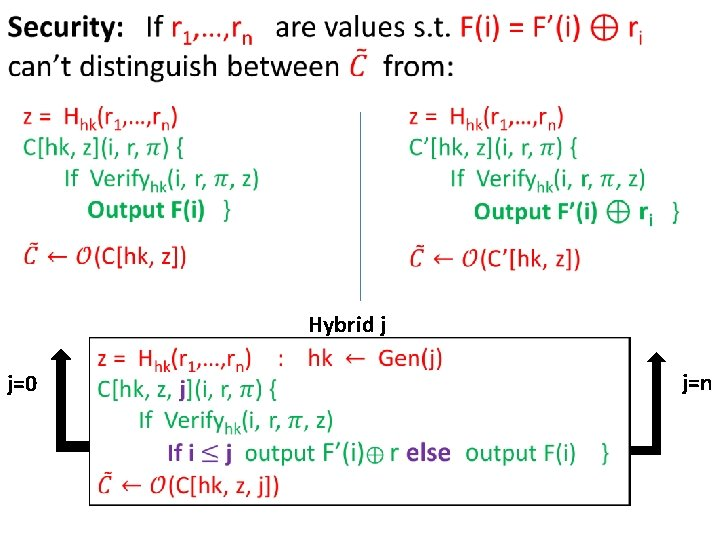Hybrid j j=0 j=nHybrid j +. 5 SSB hash key hk computationally hides binding index Hybrid j+1Constructing SSB Hash • Relies on a combination of fully-homomorphic enc (FHE) and Merkle Trees. r 0 r 1 r 2 r 3 r 4 r 5 r 6 r 7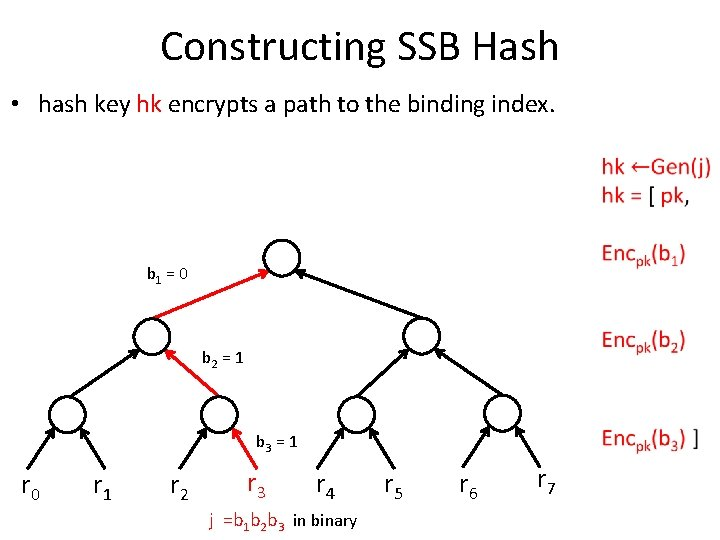Constructing SSB Hash • hash key hk encrypts a path to the binding index. b 1 = 0 b 2 = 1 b 3 = 1 r 0 r 1 r 2 r 3 r 4 j =b 1 b 2 b 3 in binary r 5 r 6 r 7Constructing SSB Hashing associates ctext with each node, output root • Leafs are encryptions of data bits (randomness 0 s) • Nodes at level t: homomorphically get an encryption of the data of left or right child depending on bit bt. [r 3] [r 7] [r 3] [r 1] r 0 [r 5] [r 3] r 1 r 2 r 3 r 4 [r 7] r 5 r 6 r 7Constructing SSB Hash • To open location i, give ciphertexts for all sibling on path from root to i. • To verify, recompute root. [r 3] [r 7] [r 3] [r 1] r 0 [r 5] [r 3] r 1 r 2 r 3 r 4 [r 7] r 5 r 6 r 7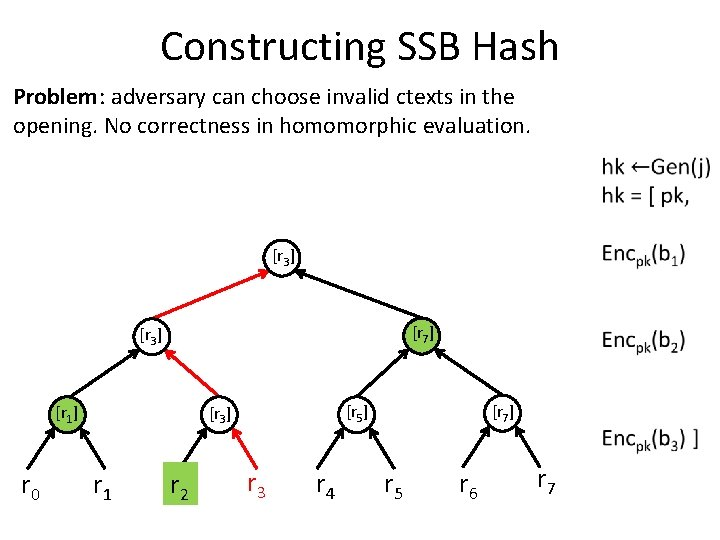Constructing SSB Hash Problem: adversary can choose invalid ctexts in the opening. No correctness in homomorphic evaluation. [r 3] [r 7] [r 3] [r 1] r 0 [r 5] [r 3] r 1 r 2 r 3 r 4 [r 7] r 5 r 6 r 7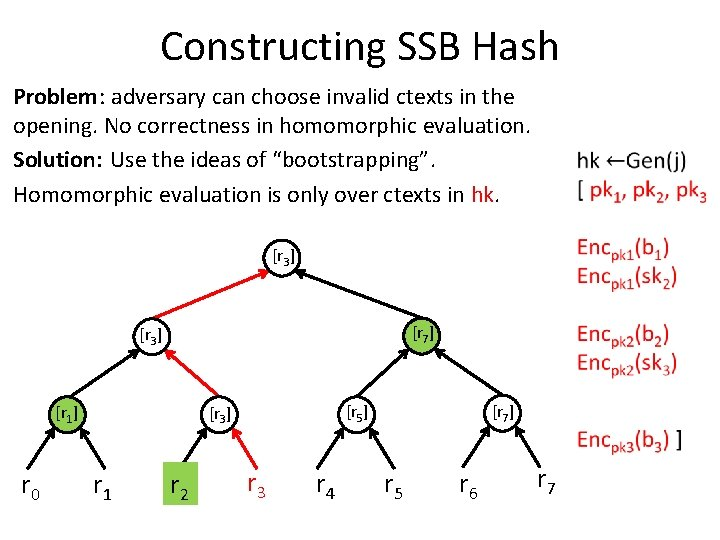Constructing SSB Hash Problem: adversary can choose invalid ctexts in the opening. No correctness in homomorphic evaluation. Solution: Use the ideas of “bootstrapping”. Homomorphic evaluation is only over ctexts in hk. [r 3] [r 7] [r 3] [r 1] r 0 [r 5] [r 3] r 1 r 2 r 3 r 4 [r 7] r 5 r 6 r 7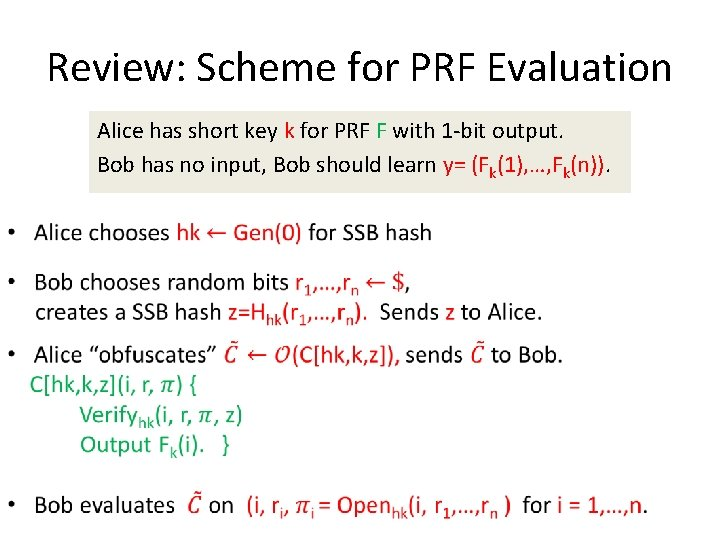Review: Scheme for PRF Evaluation • Alice has short key k for PRF F with 1 -bit output. Bob has no input, Bob should learn y= (Fk(1), …, Fk(n)).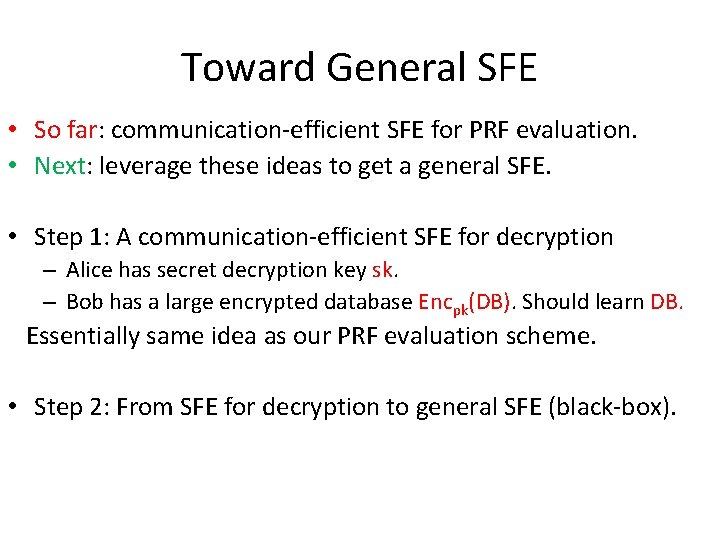Toward General SFE • So far: communication-efficient SFE for PRF evaluation. • Next: leverage these ideas to get a general SFE. • Step 1: A communication-efficient SFE for decryption – Alice has secret decryption key sk. – Bob has a large encrypted database Encpk(DB). Should learn DB. Essentially same idea as our PRF evaluation scheme. • Step 2: From SFE for decryption to general SFE (black-box).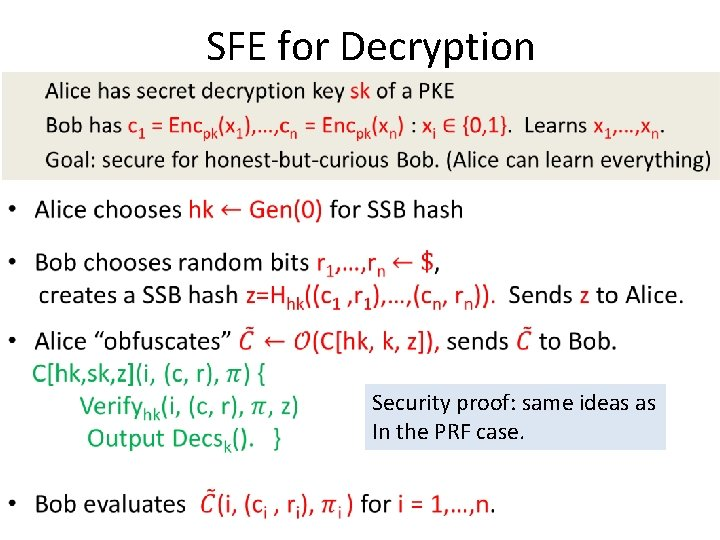SFE for Decryption • Security proof: same ideas as In the PRF case.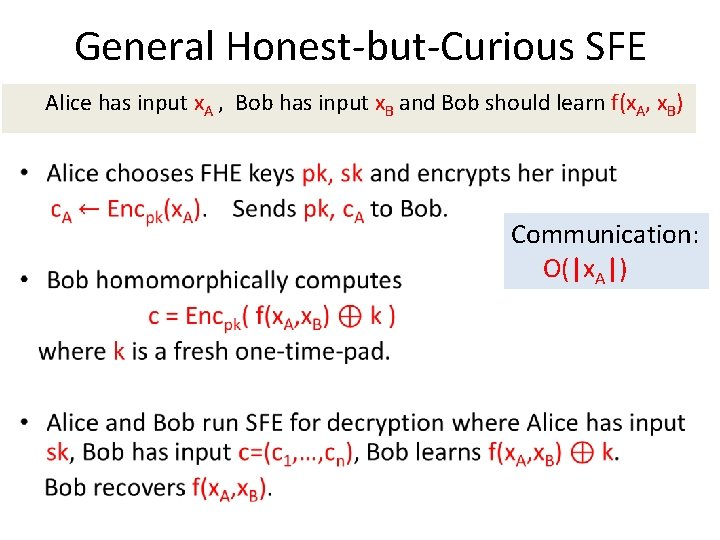General Honest-but-Curious SFE Alice has input x. A , Bob has input x. B and Bob should learn f(x. A, x. B) • Communication: O(|x. A|)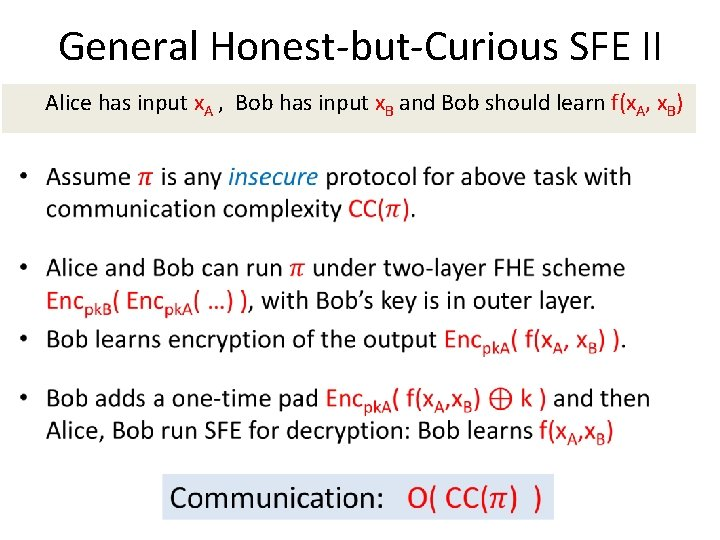General Honest-but-Curious SFE II Alice has input x. A , Bob has input x. B and Bob should learn f(x. A, x. B) •Summary: Positive Results • In the honest-but-curious setting, communication complexity of SFE matches that of insecure protocols (security is free). • Same ideas give a communication efficient protocol in the malicious setting in the common random string (CRS) model. – The simulator can choose CRS after knowing input/output of corrupted party.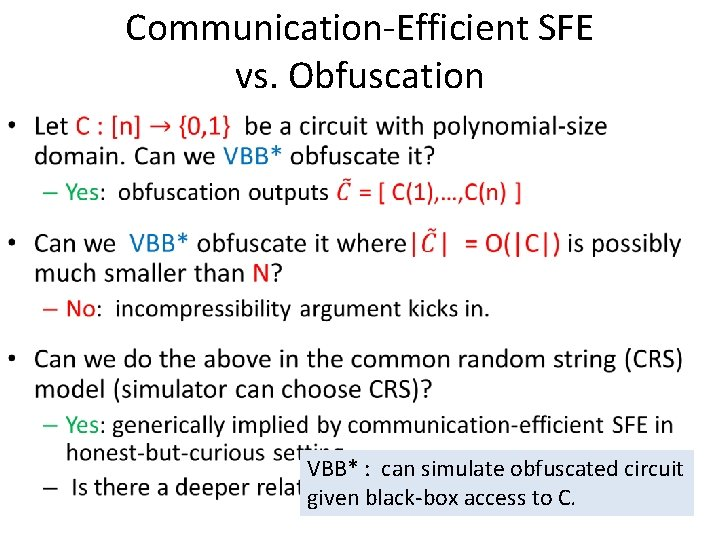Communication-Efficient SFE vs. Obfuscation • VBB* : can simulate obfuscated circuit given black-box access to C.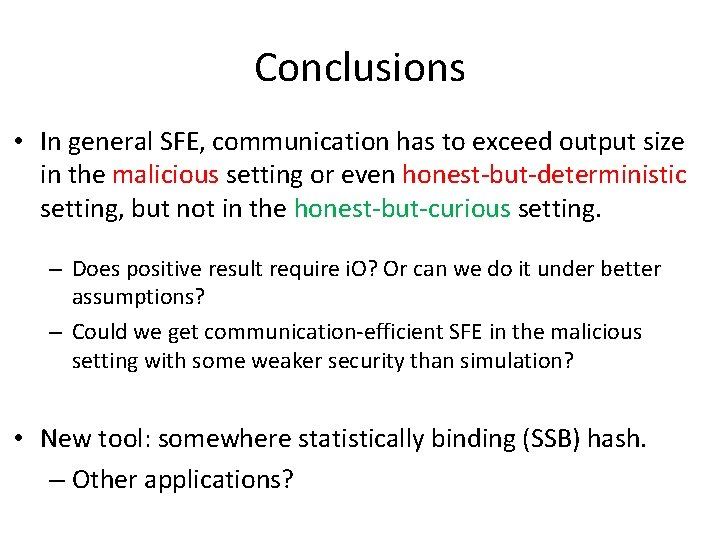Conclusions • In general SFE, communication has to exceed output size in the malicious setting or even honest-but-deterministic setting, but not in the honest-but-curious setting. – Does positive result require i. O? Or can we do it under better assumptions? – Could we get communication-efficient SFE in the malicious setting with some weaker security than simulation? • New tool: somewhere statistically binding (SSB) hash. – Other applications?